# 秃尾河流域径流量时空演变特征Temporal and Spatial Evolution Characteristics of Runoff in Tuwei River Basin

DOI: 10.12677/AG.2019.911120, PDF, HTML, XML, 下载: 335  浏览: 652  科研立项经费支持

Abstract: As actual water flow in the Middle Yellow River basin has been decreasing since the 1970s, the spatio-temporal evolution characteristics of the runoff also produce new changes. Such changes will inevitably affect the development and utilization of water resources, therefore influence the healthy, rapid development of the economy along the river. This study analyses the variability of seasonal and annual runoff in the upper reaches of the Tuwei River at Gaojiabao hydrological station from 1967 to 2012 as well as Gaojiachuan hydrological station during 1956 to 2012 in the downstream. The methods of Gini coefficient, PW-MK trend test, MK-P abrupt test and Pettit t test were employed to explain the characteristics of the seasonal and annual change of runoff. The results indicated that: the Gini coefficient of runoff in both upstream and downstream is more than 0.8, annual distribution is highly uniform, and the upstream homogeneous phenomenon is more obvious. Annual and seasonal runoff shows significant decreasing trend and mutation features. The change points of upstream runoff occurred in 1984 and 1997. The notable abrupt variation of runoff in the lower reaches was observed in 1979 and 1996. At the same time, the downstream runoff decreased more than the upstream on the 5%, 50%, and 95% frequencies, and the 95% frequency runoff decreased the most. Compared to the contrast period the impact proportion of the human activities on runoff in human activity intensity low period for the upper and lower reaches was 70.01%, 82.56%. The impact proportion was 69.37%, 77.14%, respectively in human activity intensity higher period. Human activities such as large-scale water and soil conservation measure and high intensity of coal mining have become a major factor for the change in annual runoff in both upper and lower reaches of the Tuwei River.

1. 引言

2. 流域概况

3. 数据及研究方法

3.1. 数据来源

3.2. 数据处理方法

3.2.1. 径流基尼计算

$径流\text{GI}系数=1-\underset{i-1}{\overset{n}{\sum }}\left({X}_{i}-{X}_{i-1}\right)\left({Y}_{i}+{Y}_{i-1}\right)$ (1)

3.2.2. MK-P检验方法

MK-P方法由Charles Rouge  在Mann-Kendall法和Pettit检验方法的耦合的基础上，通过定义A(n × n)矩阵，主要通过对比 $s\left(1,\tau \right)$$k\left(\tau \right)$ 变化，识别时间序列发生趋势变化和跳跃变化的确切时间，其原理步骤如下：

1) Mann-Kendall法统计量s和Pettit耦合，详见式(2)，且对于Pettit法统计量T表示(3)。

2) 判定趋势和跳跃变化。构造矩阵A，详见式(4)，使其满足式(2)。当存在突变时， $s\left(1,\tau \right)$$s\left(\tau +1,n\right)$ 之间没有相关关系，反之发生趋势变化时，两者存在相关关系。为了便于比较 $s\left(1,\tau \right)$$s\left(\tau +1,n\right)$，引入时段d，根据式(6)计算 $k\left(\tau ,d\right)$，使其Tc值。将(Tc，d)代入式(7)，当d > D时，该序列发生了突变，反之趋势变化。

$s=\underset{1c\le i\le j\le \tau }{\sum }\mathrm{sgn}\left({x}_{j}-{x}_{i}\right)+k\left(\tau \right)+\underset{\tau +1\le i\le j\le n}{\sum }\mathrm{sgn}\left({x}_{j}-{x}_{i}\right)=s\left(1,\tau \right)+k\left(\tau \right)+s\left(\tau +1,n\right)$ (2)

$T=\mathrm{arg}\underset{1\le \tau \le N}{\mathrm{max}}\left\{|k\left(\tau \right)|\right\}$ (3)

${a}_{ij}=\left\{\begin{array}{l}0\text{if}\text{\hspace{0.17em}}j\le i\\ \mathrm{sgn}\left({x}_{j}-{x}_{i}\right)\text{if}\text{\hspace{0.17em}}j>i\end{array}$ (4)

$k\left(\tau ,d\right)=\underset{i=1}{\overset{\tau +d}{\sum }}\underset{j=\tau +1}{\overset{n}{\sum }}{a}_{ij}$ (5)

${T}_{c}=\mathrm{arg}\mathrm{max}\left\{|k\left(\tau ,d\right)|\right\}$ (6)

$s=s\left(1,{T}_{c}\right)+k\left({T}_{c},d\right)+s\left({T}_{c}+d+1,n\right)$ (7)

3.2.3. SCRAQ法

SCRAQ法  在变点识别的基础上，分离基准期和不同人类活动时期；然后以时间为自变量，累计径流、降水、蒸发量为因变量，根据不同时段累计量斜率差值，计算气候变化和人类活动对径流的影响。采用时间这一客观变量为自变量，累计值为因变量，与双累计曲线相比，有效降低了两者变化率一致导致的误差，这一方法在黄河流域，尤其是皇甫川流域得到了较好的验证。计算步骤如下：

${R}_{KR}=100×\left({K}_{Ra}-{K}_{Rb}\right)/{K}_{Rb}$ (8)

${R}_{KP}=100×\left({K}_{Pa}-{K}_{Pb}\right)/{K}_{Pb}$ (9)

${R}_{KEO}=100×\left({K}_{EOa}-{K}_{EOb}\right)/{K}_{EOb}$ (10)

${C}_{P}=100×{R}_{KP}/{R}_{KR}$ (11)

${C}_{EO}=100×{R}_{KEO}/{R}_{KR}$ (12)

${C}_{H}=100-{C}_{P}-{C}_{E}{}_{O}$ (13)

4. 径流时空演变特征

4.1. 径流年内分配均匀度特征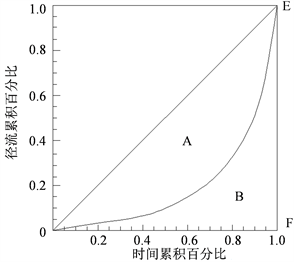Figure 1. The variation of runoff Gini coefficientFigure 2. The variation of runoff and Gini coefficient of the Tuwei River

4.2. 径流年际变化趋势及突变分析

PW-MK检验方法  是为了剔除时间序列的自相关性，具体步骤如下：① 计算序列Xt的在δ置信水平下的一阶自相关系数r1，并采用双侧检验进行r1的显著性检验 ；② 根据式(14)求出不具有自相关性的时间序列。最后，应用MK方法来检验重组序列趋势变化的显著性。

${{X}^{\prime }}_{t}={X}_{t}-{r}_{1}{X}_{t-1}$ (14)Table 1. Results of trend tests for annual runoff variables by PW-MK in 5 time series in the upper and lower reaches of the Tuwei River basin(a)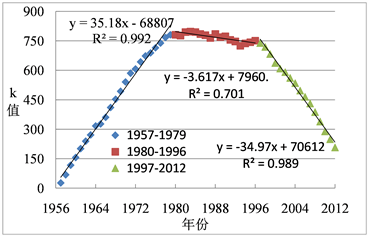(b)

Figure 3. (a) Change of k value calculated by Pettit t method in Gaojiabao hydrologic station; (b) Change of k value calculated by Pettit t method in Gaojiachuan hydrologic station

4.3. 不同时期游径流变化程度分析Table 2. The relative changes represented by daily runoff curves in high, median and low flows in 5 time series in the upper and lower reaches of the Tuwei River basin

4.4. 不同时期人类活动对径流影响评价

4.4.1. 不同时期人类活动对径流影响的分离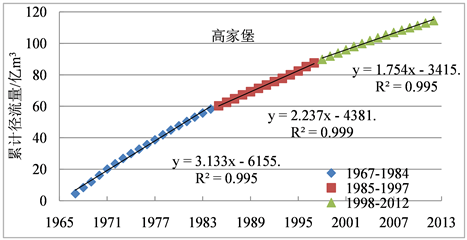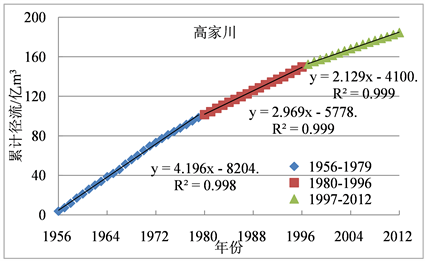Figure 4. Relationships between year and cumulative runoff of Gaojiabao and Gaojiachuan hydrologic stationTable 3. The proportion and change of cumulative runoff, precipitation and potential evaporation in different periodsTable 4. Contributions proportion of climate change and human activities to the runoff changes at different periods in the upper and lower reaches of the Tuwei River basin

4.4.2. 人类活动对径流变化的影响

1) 水土保持对径流变化的影响。秃尾河上、下游径流量h1期显著减少可能与水土保持，尤其是林草地面积的快速增加有关。70年代末期流域开始大规模水土保持工作，水土保持面积由1979年的229.15 km2增加到1996的1140.93 km2，占流域面积比例由7%上升到35.1%。林草面积所占比例由1979年的5.82%增加为1996年的32.58% 。黄河水利委员会绥德水土保持试验站观测资料表明，林地盖度为30%、50%、70%，分别减少地表径流53%、86%、94%。另外，上游径流突变时间滞后于下游，可能与上游水土保持措施开始实施时间、水土保持未达到预期效果有关。

2) 煤矿开采对径流变化的影响。上世纪90年代，尤其是1996年之后，秃尾河流域煤矿开采量和采空区面积不断增加，详见图5，采煤塌陷形成的地裂缝一方面使降雨补给向下渗漏，消减地表径流。另一方面，导水裂隙带发育至第四系含水层底部，造成潜水位大幅度下降，泉流量减少，甚至干涸，引起河流的侧向补给来源明显减少 。由表4可知，相对于h1期流域上、下游h2期(主要为煤炭开采)，人类活动对径流的影响比例分别为95.74%和90.73%。

3) 地下水开发利用对径流的影响。秃尾河流域地下水开发是导致秃尾河径流减少的另一原因。秃尾河径流的68%  来自基流的补给。随着流域经济快速发展，流域用水量不断增加。地下水的供水量由2006年的8.31万m3，增加到2010年的8.84万m3，并持续增加。地下水开采过程中宜采用引泉开采，而实际多选用分散井或集中水源地开采。地下水开采量的增加和不合理的开发利用，消减地下水对径流的补给。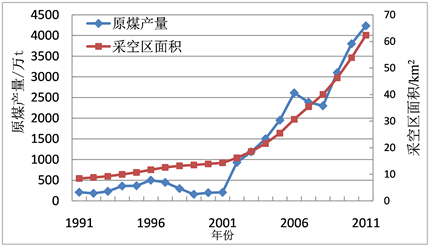Figure 5. The output of raw coal and the actual area of underground goaf during 1991-2011

4) 水利工程建设对径流变化的影响。秃尾河流域一系列水利工程设施的建成和运行，在改变径流时间分布分配的同时，也影响到水文监测断面的实测径流量。

5. 结论

1) 秃尾河上下游径流基尼系数值超过0.8，径流年内分布高度均匀，上游分布更均匀。年径流基尼系数有略微上升趋势，径流年际随时间分布的稳定性有增强趋势，夏季径流量高于其他季节，除夏季外，其他4个时段上游下降率明显高于下游，是径流年内变化趋于均匀化，上游分布更均匀，年际径流稳定性增强的重要原因之一。

2) 秃尾河上、下游5个时段(全年、春季、夏季、秋季、冬季)年径流呈现显著下降趋势，且具有显著的突变特征，上游径流量以1984和1997年为突变点，下游突变发生在1979和1996年。与基准期相比，h1期流域上、下游年径流量5%频率分别减少25%~30%、30%；50%频率减少30%~35%、20%~25%，95%频率分别减少30%~35%、15%~20%；h2期上、下游年径流量在5%频率分别减少40%~45%，50%~55%，50%频率分别减少40%~45%，45%~50%，95%频率减少40%~45%，45%~50%。与h1期相比，上游h2期径流量减少是前期的1.67倍，下游是前期的2.11倍。同一时期不同频率下，上、下游年径流量降幅均大于上游，且95%频率年径流量减少幅度最大。

3) 与基准期相比，人类活动较低期上、下游人类活动对径流变化的影响比例分别为70.01%，82.56%，人类活动较高期人类活动对上、下游径流的影响比例分别为69.37%，77.14%。与人类活动较低期相比，人类活动较高期对上、下游径流的影响比例分别为95.74%和90.73%。大规模水土保持和高强度煤炭开采是影响秃尾河径流减少的主要原因，地下水开发利用、水利工程建设和气候变化的影响也不可忽视。

  Birsan, M.-V., Molnar, P., et al. (2005) Stream Flow Trends in Switzerland. Journal of Hydrology, 314, 312-329. https://doi.org/10.1016/j.jhydrol.2005.06.008  Omar, I., Aziz, A. and Burn, D.H. (2006) Trends and Variability in the Hydrological Regime of the Mackenzie River Basin. Journal of Hydrology, 319, 282-294. https://doi.org/10.1016/j.jhydrol.2005.06.039  李勃, 穆兴民, 高鹏, 等. 1956-2017年黄河干流径流量时空变化新特征[J]. 水土保持研究, 2019, 26(6): 120-126+132.  胡慧杰, 崔凯, 曹茜, 等. 黄河近百年径流演变特征分析[J]. 人民黄河, 2019, 41(9): 14-19.  胡春宏, 王延贵, 张燕菁, 等. 中国江河水沙变化趋势与主要影响因素[J]. 水科学进展, 2010, 21(4): 524-532.  范念念, 吴保生, 田富强. 沙地地区水文过程及模拟——以秃尾河流域为例[J]. 水文, 2013, 33(1): 12-17.  刘智勇, 张鑫, 李小冰. 气候变化对秃尾河流域径流量的影响[J]. 干旱地区农业研究, 2001, 29(6): 184-190.  白桦, 穆兴民, 王双银. 水土保持措施对秃尾河径流的影响[J]. 水土保持研究, 2010, 17(1): 41-44.  孙天青, 张鑫, 梁学玉, 等. 秃尾河径流特性及人类活动对径流的影响分析[J]. 人民长江, 2010, 41(8): 47-50.  赵琳琳. 基于PW-MK及小波分析的辽阳降水特征研究[J]. 灌溉排水学报, 2017, 36(9): 97-101.  Rougé, C., Ge, Y. and Ca, X.M. (2013) Detecting Gradual and Abrupt Changes in Hydrological Records. Advances in Water Resources, 53, 33-44. https://doi.org/10.1016/j.advwatres.2012.09.008  Kong, B., Huang, S., Ma, L., et al. (2018) Spatio-Temporal Changes in Potential Evaporation and Possible Causes Based on SCRAQ Method: A Case Study in the Wei River Basin, China. Journal of Coastal Research, 84, 94-102. https://doi.org/10.2112/SI84-014.1  谢贤群, 王菱. 中国北方近50年潜在蒸发的变化[J]. 自然资源学报, 2007, 22(5): 683-691.  穆兴民, 高鹏, 巴桑赤烈, 等. 应用流量历时曲线分析黄土高原水利水保措施对河川径流的影响[J]. 地球科学进展, 2008, 23(4): 382-389.  刘德地, 陈晓宏. 一种区域用水量公平性的评估方法[J]. 水科学进展, 2008, 19(2): 268-272.  Anderson, R.L. (1942) Distribution of the Serial Correlation Coefficients. Annals of Mathematical Statistics, 13, 1-13. https://doi.org/10.1214/aoms/1177731638  Wang, S., Yan, M., Yan, Y., et al. (2012) Contributions of Climate Change and Human Activities to the Changes in Runoff Increment in Different Sections of the Yellow River. Quaternary International, 282, 66-77. https://doi.org/10.1016/j.quaint.2012.07.011  Pettitt, A.N. (1979) A Non-Parametric Approach to the Change-Point Problem. Applied Statistics, 28, 126-135. https://doi.org/10.2307/2346729  周旭. 变化环境下秃尾河径流演变规律研究[D]: [硕士学位论文]. 杨凌: 西北农林科技大学, 2012.  张发旺, 赵红梅, 宋亚新, 等. 神府东胜矿区采煤塌陷对水环境影响效应研究[J]. 地球学报, 2007, 28(6): 521-527.  钱云平, 蒋秀华, 金双彦, 等. 黄河中游黄土高原区河川基流特点及变化分析[J]. 地球科学与环境学报, 2004, 26(2): 88-91.